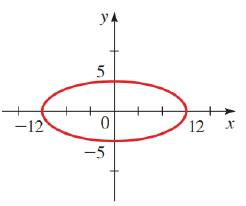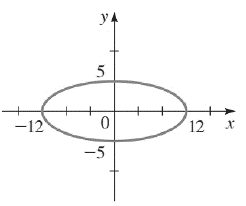Chapter 12.CR, Problem 38E### Algebra and Trigonometry (MindTap ...

4th Edition
James Stewart + 2 others
ISBN: 9781305071742

#### Solutions

Chapter
Section### Algebra and Trigonometry (MindTap ...

4th Edition
James Stewart + 2 others
ISBN: 9781305071742
Textbook Problem

# 37-42 Finding the Equation of a Conic Find an equation for the conic whose graph is shown.To determine

To find:

An equation of the conic whose graph is given below.Explanation

Given:

The major axis is 12 and the minor axis is 5.

Approach:

An equation of an ellipse is x2a2+y2b2=1

Calculation:

The major axis is 12 and the minor axis is 5.

So,

2a=12a=6

### Still sussing out bartleby?

Check out a sample textbook solution.

See a sample solution

#### The Solution to Your Study Problems

Bartleby provides explanations to thousands of textbook problems written by our experts, many with advanced degrees!

Get Started